A 38MW Turbine Aerodynamic Calculation: NUMECA vs CFX

Taking a 38MW steam turbine as an example, two software applications are used for aerodynamic analysis to calculate the settings of the boundary condition. Flow parameters: total inlet temperature 70.125 ° C, total inlet pressure 31.346 kPa, static pressure at outlet 8.89 kPa, and inlet airflow angle 0 degree.

CFX's residual standard is set to 10 ^ -5, the number of iteration steps is 1000, with a 40-core CPU, are calculated in parallel; NUMECA's residual standard is set to 10 ^ -6.5, the number of iteration steps is 3000, single-core calculation and single-precision calculation. The comparison of the residual curve is shown in Figure 1, the comparison of the impeller outlet pressure monitoring curve is shown in Figure 2, and the comparison of the isentropic efficiency curve is shown in Figure 3.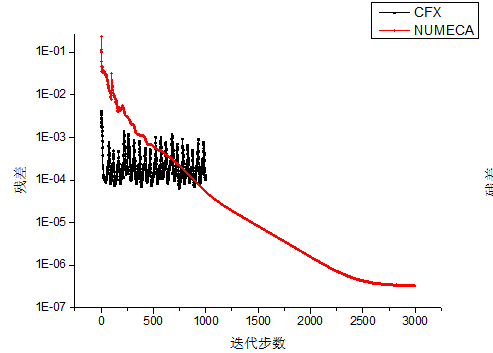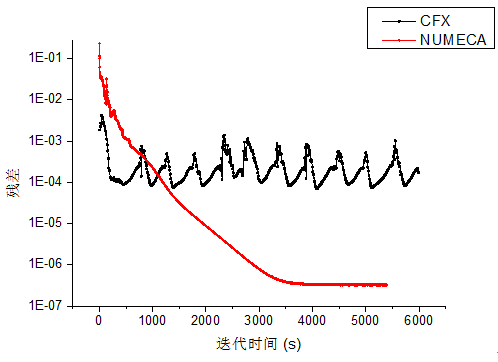Figure1Comparison chart of residual curve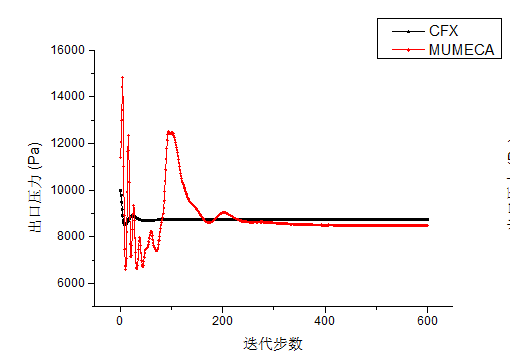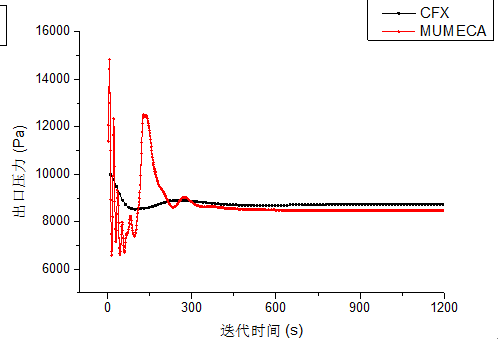Figure2Comparison chart of outlet pressure monitoring curve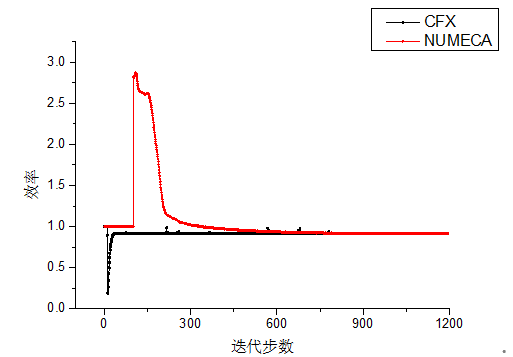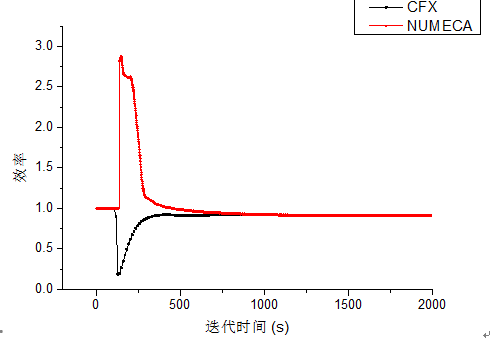Figure3Efficiency curve comparison chart

It can be seen that under the same calculation conditions, the residual error of NUMECA when reaching the convergence standard is about 10 ^ -6.5, the calculation accuracy is high, and the convergence of its residual curve is better than CFX; meanwhile, the calculation time of NUMECA is much less In CFX, as it takes about an hour. Also, the NUMECA calculation results can be easily connected to Tecplot for post-processing. Therefore, NUMECA should be recommended in the aerodynamic simulation of steam turbines.